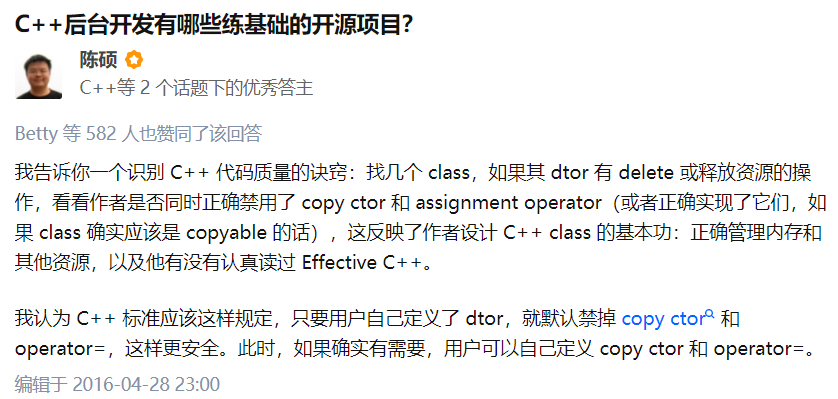# 左值与右值

• 左值对应于一个实实在在的内存位置，右值只是临时的对象，它的内存对程序来说只能读不能写。

## 基本概念

int i = 10;
i = 5;
int a = i;
a = i;


10 = i; // error


## 引用

// int& b = 5;  // can't reference rvalue
int& c = i;     // allowed


## 函数返回值

int GetValue() {
return 5;
}
i = GetValue();
GetValue() = i; // error


int& GetLValue() {
static int value = 10;
return value;
}
i = GetLValue(); // true
GetLValue() = i; // true


## 函数参数

void SetValue(int value) {}

void SetLValue(int& value) {}

SetValue(i);
SetValue(5);

SetLValue(i);
SetLValue(5); // error


## Const

const int & d = 5;

void SetConstValue(const int& value) {}
SetConstValue(i);
SetConstValue(5);


## 右值引用

void PrintName(const std::string& name) {
std::cout << "[lvalue] " << name << std::endl;
}
void PrintName(const std::string&& name) {
std::cout << "[rvalue] " << name << std::endl;
}

std::string firstName = "Yan";
std::string lastName = "Chernikov";
std::string fullName = firstName + lastName;

PrintName(fullName);
PrintName(firstName + lastName);


[lvalue] YanChernikov
[rvalue] YanChernikov


# 移动语义

## 例子

#include <iostream>
#include <cstring>

class String {
public:
String() = default;
String(const char* string) {
printf("Created!\n");
m_Size = strlen(string);
m_Data = new char[m_Size];
memcpy(m_Data, string, m_Size);
}

String(const String& other) {
printf("Copied!\n");
m_Size = other.m_Size;
m_Data = new char[m_Size];
memcpy(m_Data, other.m_Data, m_Size);
}

~String() {
delete[] m_Data;
}

void Print() {
for (uint32_t i = 0; i < m_Size; ++i)
printf("%c", m_Data[i]);

printf("\n");
}
private:
char* m_Data;
uint32_t m_Size;
};

class Entity {
public:
Entity(const String& name)
: m_Name(name) {}
void PrintName() {
m_Name.Print();
}
private:
String m_Name;
};

int main(int argc, const char* argv[]) {
Entity entity(String("Cherno"));
entity.PrintName();

return 0;
}


Created!
Copied!
Cherno


## 移动构造函数

    String(String&& other) {
printf("Moved!\n");
m_Size = other.m_Size;
m_Data = other.m_Data;
other.m_Data = nullptr;
other.m_Size = 0;
}

~String() {
printf("Destroyed!\n");
delete[] m_Data;
}

Entity(String&& name)
: m_Name(name) {}

// Entity(const String& name)
//     : m_Name(name) {}



Created!
Copied!
Destroyed!
Cherno
Destroyed!


Entity(String&& name)
:m_Name((String&&)name) {}


Entity(String&& name)
:m_Name(std::move(name)) {}


Created!
Moved!
Destroyed!
Cherno
Destroyed


## 移动赋值运算符

int main(int argc, const char* argv[]) {
String apple = "apple";
String orange = "orange";

printf("apple: ");
apple.Print();
printf("orange: ");
orange.Print();

apple = std::move(orange);

printf("apple: ");
apple.Print();
printf("orange: ");
orange.Print();
return 0;
}


    String& operator=(String&& other) {
printf("Moved\n");
if (this != &other) {
delete[] m_Data;

m_Size = other.m_Size;
m_Data = other.m_Data;

other.m_Data = nullptr;
other.m_Size = 0;
}
return *this;
}


Created!
Created!
apple: apple
orange: orange
Moved
apple: orange
orange:
Destroyed!
Destroyed!


# C++ 三/五法则# 参考资料

Cherno C++视频教程

C++ 三/五法则 - 阿玛尼迪迪 - 博客园 (cnblogs.com)

posted @ 2022-03-17 19:57  zhangyi1357  阅读(3337)  评论(4编辑  收藏  举报